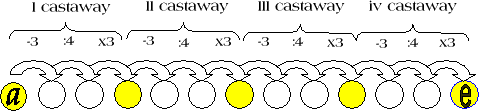#### You may also like### Sixational

The nth term of a sequence is given by the formula n^3 + 11n . Find the first four terms of the sequence given by this formula and the first term of the sequence which is bigger than one million. Prove that all terms of the sequence are divisible by 6.### Telescoping Functions

Take a complicated fraction with the product of five quartics top and bottom and reduce this to a whole number. This is a numerical example involving some clever algebra.### Sums of Squares and Sums of Cubes

An account of methods for finding whether or not a number can be written as the sum of two or more squares or as the sum of two or more cubes.

# Old Nuts

##### Age 16 to 18 Challenge Level:

Super solutions to 'Old Nuts' came in from Vassil Vassilev of Lawnswood High School, Leeds, Hyeyoun Chung of St Paul' Girls's School London and Elizabeth Whitmore of Madras College, St Andrew's.

Here is Vassil's diagram and Hyeyoun's solution:.Let us call the total number of coconuts in the beginning $a$, then the number of coconuts in the remaining pile $b$, then the number of nuts in the next pile of remaining nuts $c$, and so on, the number of nuts in the last pile being $e$.

First of all we can say that: $a - 3$ is a multiple of 4.

We can also say that:

$b = 3(a -3 )/4$
$c = 3(b -3 )/4$
$d = 3(c -3 )/4$
$e = 3(d -3 )/4$

Then, as each pile has 3 nuts taken away from it and then is divided into 4 equal piles, we can say that:

$a - 3$ is a multiple of 4
$b - 3$ is a multiple of 4
$c - 3$ is a multiple of 4
$d - 3$ is a multiple of 4

Also, as each pile except for $a$ has been created by taking 3/4 of the previous pile minus 3, we can also say that:

$b$ is a multiple of 3 and $b - 3$ is a multiple of 4 and 3
$c$ is a multiple of 3 and $c - 3$ is a multiple of 4 and 3
$d$ is a multiple of 3 and $d - 3$ is a multiple of 4 and 3
$e$ is a multiple of 4 and 3, as the castaways were able to divide it exactly into four equal piles.

The least number of coconuts the castaways could have ended with will also give you the least number of coconuts they could have started with. The number of coconuts the castaways ended up with, $e$, is a common multiple of 4 and 3, which means it is a multiple of 12. The smallest common multiples of 4 and 3 are 12, 24, 36, 48, 60, 72, ...

Trying out these numbers one by one, the smallest multiple of 12 which satisfies the above conditions is 72. This is shown below:

When $e = 12$, $d = 4e/3+ 3 = 19$. However $d$ needs to be a multiple of 3 and so this will not work.
When $e = 24$, $d = 4e/3+ 3 = 35$. Again, this will not work.
When $e = 36$, $d = 4e/3+ 3 = 51$. This seems to work. However: see what follows.
When $d = 51$, $c = 4d/3+ 3 = 71$. As $c$ also needs to be a multiple of 3, this will not work either.

The same thing occurred with all the other multiples of 12. However, with 72:
When $e = 72$, $d = (4\times 72)/3 + 3 = 99$.
When $d = 99$, $c = 4d/3 + 3 = 135$.
When $c = 135$, $b = 4c/3 + 3 = 183$.
When $b = 183$, $a = 4d/3 + 3 = 247$.

Here, $b$, $c$, and $d$ are all multiples of 3, and $b - 3$, $c - 3$, and $d - 3$ are all multiples of 3 and 4. As $a$ does not have to be a multiple of either 3 or 4, this works.

Therefore, the least number of coconuts the castaways could have started with is 247.

You may like to apply the methods of modulus arithmetic and send in a Further Inspiration on this problem. See the NRICH articles on modulus arithmetic.